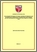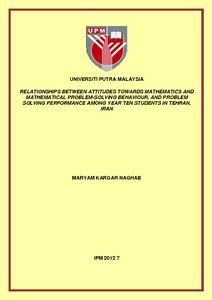# Relationships between attitudes towards mathematics and mathematical problem-solving behaviour, and problem solving performance among year ten students in Tehran, Iran

## Citation

Kargar Naghab, Maryam (2012) Relationships between attitudes towards mathematics and mathematical problem-solving behaviour, and problem solving performance among year ten students in Tehran, Iran. Masters thesis, Universiti Putra Malaysia.

## Abstract

A descriptive correlational research design was conducted to investigate the correlation between mathematical problem solving performance and strategy with mathematics attitudes and mathematical problem solving behaviours. The subjects of this study were 150 Mathematics and Physics year 10 students from Tehran city which is capital city of Iran. Among 22 regions of Tehran city, 3 regions were selected randomly by using fishbowl method. Ten high schools from these three regions participated in this study. Three instruments were used in this study namely, the Mathematical Problem Solving Test, Mathematics Attitudes Scale, and Mathematical Problem Solving Behaviours Scale. The data was analysed by using Pearson correlation to show the correlation among variables. The study shows that there is high positive significant correlation between students’ mathematical problem solving performance and students’ mathematics attitudes. In addition, a high positive significant correlation was found between students’ mathematical problem solving behaviour and mathematical problem solving performance. The results also indicate that there is a high positive significant correlation between students’mathematics attitudes and students’ mathematical problem solving behaviour with executing the appropriate mathematical problem solving strategies. These findings indicated that problem solving performance is correlated with mathematics attitudes and problem solving behaviour, hence mathematical problem solving ability can be enhanced with nurturing positive attitudes toward mathematics learning and also students’ behaviour in problem solving.Preview
PDF
IPM 2012 7R.pdfView Item